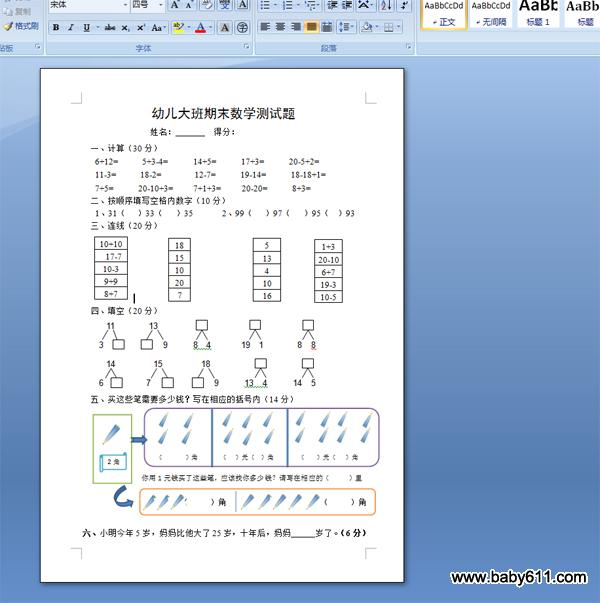幼儿园大班数学上期期末测试题幼儿大班期末数学测试题

姓名：         得分：

一、计算（30分）

6+12=      5+3-4=      14+5=      17+3=      20-5+2=

11-3=      18-2=        12-7=      19-14=      18-18+1=

7+5=      20-10+3=     7+1+3=     20-20=      8+3=

二、按顺序填写空格内数字（10分）

1、31（   ）33（   ）35       2、99（   ）97（   ）95（  ）93

三、连线（20分）

10+10

四、填空（20分）

11        13

3              9      8  4       19  1        8  8

14         15         18

6          7               9      13  4      14  5

五、买这些笔需要多少钱？写在相应的括号内（14分）

六、小明今年5岁，妈妈比他大了25岁，十年后，妈妈      岁了。（6分）

• 幼儿园试卷分类
• 将此试卷分享到：
• 大班试卷最新更新